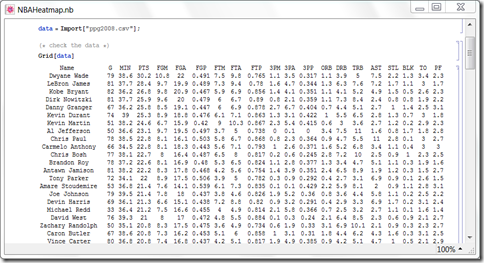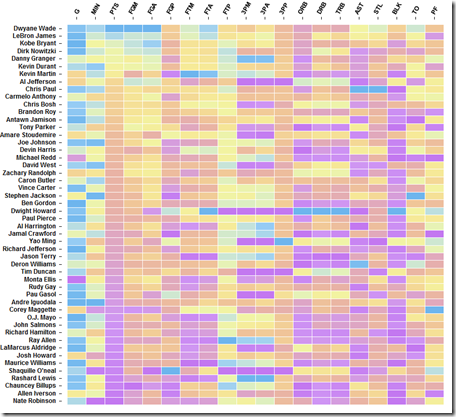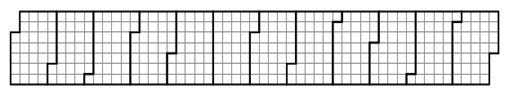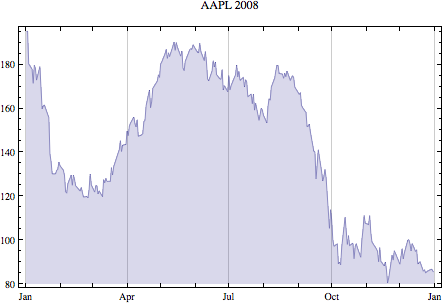## Jan 26, 2010

### More on Heatmap

data = Import["ppg2008.csv"];

Grid[data]numbers = data[[2 ;; All, 2 ;; All]]; (* this is the data for heatmap *)
playernames = data[[2 ;; All, 1]]; (* for the labels *)
statnames = data[[1, 2 ;; All]]; (* for the labels *)

Then we need to scale the each column separately to [0,1].

newnumber = Transpose[Rescale[#] & /@ (Transpose[numbers])];

Then you can generate a simple heatmap simply by ArrayPlot[newnumber].

Of course, we like to add the labels with FrameTicks option. FrameTicks->{{left,right},{bottom,top}}  mark options specified separately for each edge.

Transpose[{Range[Length[playernames]], playernames}] generate the list {{1, “player1”} … {50, “player50”}} to label the player names.

Transpose[{Range[Length[statnames]], Map[Rotate[#, 60 Degree] &, statnames]}] with this line, we can rotate the labels at the same time.

Here is the final product, click to see the full graphic.## Jan 12, 2010

### Charting time series as calendar heat maps

I haven’t updated this blog for a while. I am working on one Matlab project right now. It is kind of difficult for me to work with Matlab and Mathematica at the same time.

There is an interesting post Charting time series as calendar heat maps in R. The original idea from SAS Analysis of airline performance. I create a simple version in Mathematica. The tricky part is to generate the background grid.The code I use is from an old tutorial of Making a Calendar. For each month, the boundary is defined by 8 points, marked out by darker line. The monthly grids are shifted to the right positions to form a yearly grid. Once you figure out this part, the rest is just straightforward.

I use the stock as the example, this  is actually not the best data set for this type of visualization.Calendar heatmap: